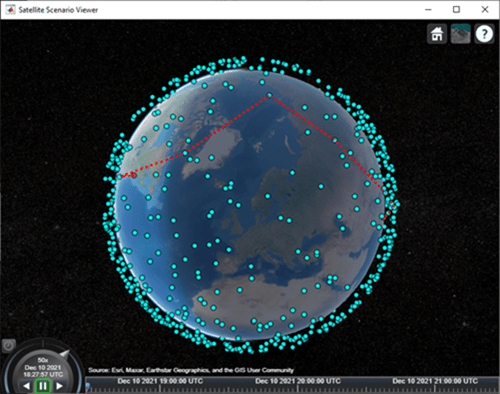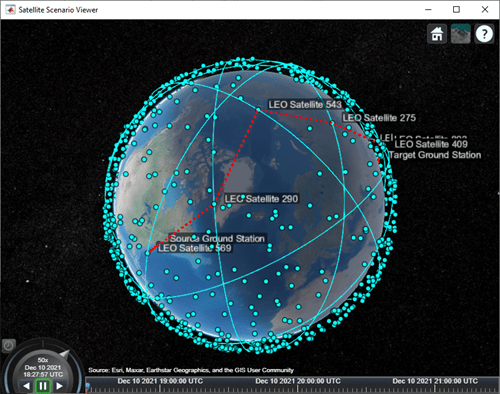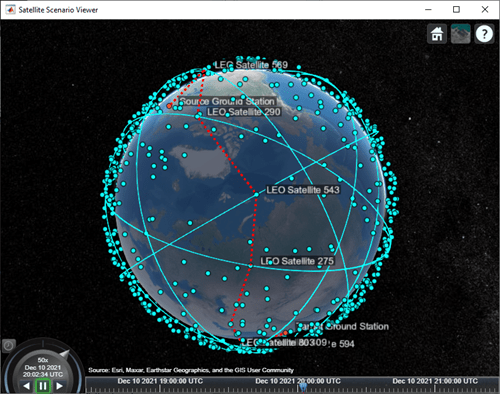# Multi-Hop Path Selection Through Large Satellite Constellation

This example shows how to determine the path through a large constellation consisting of 1,000 low-Earth orbit (LEO) satellites to gain access between two ground stations. Following this, it demonstrates how to calculate the intervals during the next 3 hour period when this path can be used.

### Create Satellite Scenario

Assume that the path through the large constellation to establish access between two ground stations must be determined as of 10 December 2021, 6:27:57 PM UTC. We must then determine the times over the next 3 hours when this path can be used. Accordingly, create a satellite scenario with the appropriate `StartTime` and `StopTime`. Set `SampleTime` to 60 seconds. Since the path must be determined only for the first time step, set `AutoSimulate` of the scenario to false to prevent it from automatically advancing through the time steps until `StopTime`. When `AutoSimulate` is false, `SimulationStatus` becomes available.

```startTime = datetime(2021,12,10,18,27,57); % 10 December 2021, 6:27:57 PM UTC stopTime = startTime + hours(3); % 10 December 2021, 9:27:57 PM UTC sampleTime = 60; % Seconds sc = satelliteScenario(startTime,stopTime,sampleTime,"AutoSimulate",false)```
```sc = satelliteScenario with properties: StartTime: 10-Dec-2021 18:27:57 StopTime: 10-Dec-2021 21:27:57 SampleTime: 60 SimulationTime: 10-Dec-2021 18:27:57 SimulationStatus: NotStarted AutoSimulate: 0 Satellites: [1×0 matlabshared.satellitescenario.Satellite] GroundStations: [1×0 matlabshared.satellitescenario.GroundStation] Viewers: [0×0 matlabshared.satellitescenario.Viewer] AutoShow: 1 ```

### Add Large Constellation of Satellites

Add the large satellite constellation from the Two-Line-Element (TLE) file `largeConstellation.tle`. The constellation consists of 1,000 LEO satellites.

```sat = satellite(sc,"largeConstellation.tle"); numSatellites = numel(sat)```
```numSatellites = 1000 ```

Add the ground stations. A multi-hop path must be established through the satellite constellation for access between the ground stations.

```gsSource = groundStation(sc,42.3001,-71.3504, ... % Latitude and longitude in degrees "Name","Source Ground Station"); gsTarget = groundStation(sc,17.4351,78.3824, ... % Latitude and longitude in degrees "Name","Target Ground Station");```

### Determine Elevation Angles of Satellites with Respect to Ground Stations

Determine the elevation angle of each satellite with respect to source and target ground stations corresponding to `StartTime`. Accordingly, use `advance` to simulate the scenario for the first time step, namely, `StartTime`. Following this, use `aer` to retrieve the elevation angle of each satellite with respect to the ground stations. Assume that for the initial routing, the elevation angle of the first satellite in the path with respect to "Source Ground Station" and the last satellite in the path with respect to "Target Ground Station" must be at least 30 degrees. Accordingly, determine the elevation angles that are greater than or equal to this value.

```% Calculate the scenario state corresponding to StartTime. advance(sc); % Retrieve the elevation angle of each satellite with respect to the ground % stations. [~,elSourceToSat] = aer(gsSource,sat); [~,elTargetToSat] = aer(gsTarget,sat); % Determine the elevation angles that are greater than or equal to 30 % degrees. elSourceToSatGreaterThanOrEqual30 = (elSourceToSat >= 30)'; elTargetToSatGreaterThanOrEqual30 = (elTargetToSat >= 30)';```

The best satellite to be used for the initial access to the large constellation is assumed to be the one that satisfies the following simultaneously:

• Has the closest range to "Target Ground Station".

• Has an elevation angle of at least 30 degrees with respect to "Source Ground Station".

```% Find the indices of the elements of elSourceToSatGreaterThanOrEqual30 % whose value is true. trueID = find(elSourceToSatGreaterThanOrEqual30 == true); % These indices are essentially the indices of satellites in sat whose % elevation angle with respect to "Source Ground Station" is at least 30 % degrees. Determine the range of these satellites to "Target Ground % Station". [~,~,r] = aer(sat(trueID), gsTarget); % Determine the index of the element in r bearing the minimum value. [~,minRangeID] = min(r); % Determine the element in trueID at the index minRangeID. id = trueID(minRangeID); % This is the index of the best satellite for initial access to the % constellation. This will be the first hop in the path. Initialize a % variable 'node' that stores the first two nodes of the routing - namely, % "Source Ground Station" and the best satellite for initial constellation % access. nodes = {gsSource sat(id)};```

### Determine Remaining Nodes in Path to Target Ground Station

The remaining nodes in the path are determined using a similar logic as what was used for determining the first satellite. If the elevation angle of the satellite in the current node is already at least 30 degrees with respect to "Target Ground Station", the next node is "Target Ground Station", thereby completing the path. Otherwise, the next node in the constellation is chosen using a logic that is similar to what was used for determining the first satellite in the path. The next node is the one that simultaneously satisfies the following:

• Has the closest range to "Target Ground Station".

• Elevation angle with respect to the satellite in the current node is greater than or equal to -15 degrees.

The elevation value of -15 degrees is chosen because the horizon with respect to each satellite in the constellation is about -21.9813 degrees. This value can be derived by assuming a spherical Earth geometry and the fact that these satellites are in near-circular orbits at an altitude of roughly 500 kilometers. Note that the spherical Earth assumption is used only for computing the elevation angle of the horizon below. The satellite scenario simulation itself assumes a WGS84 ellipsoid model for the Earth.

```earthRadius = 6378137; % meters altitude = 500000; % meters horizonElevationAngle = asind(earthRadius/(earthRadius + altitude)) - 90 % degrees```
```horizonElevationAngle = -21.9813 ```

Any satellite whose elevation angle with respect to another satellite is greater than -21.9813 degrees is guaranteed to be visible to the latter. However, choosing -15 degrees provides an adequate margin.

The subsequent nodes are continually added to the path until reaching a satellite whose elevation angle with respect to "Target Ground Station" is at least 30 degrees. After this, the final node is "Target Ground Station" itself, and the routing is complete.

```% Minimum elevation angle of satellite nodes with respect to the prior % node. minSatElevation = -15; % degrees % Flag to specify if the complete multi-hop path has been found. pathFound = false; % Determine nodes of the path in a loop. Exit the loop once the complete % multi-hop path has been found. while ~pathFound % Index of the satellite in sat corresponding to current node is % updated to the value calculated as index for the next node in the % prior loop iteration. Essentially, the satellite in the next node in % prior iteration becomes the satellite in the current node in this % iteration. idCurrent = id; % This is the index of the element in elTargetToSatGreaterThanOrEqual30 % tells if the elevation angle of this satellite is at least 30 degrees % with respect to "Target Ground Station". If this element is true, the % routing is complete, and the next node is the target ground station. if elTargetToSatGreaterThanOrEqual30(idCurrent) nodes = {nodes{:} gsTarget}; %#ok<CCAT> pathFound = true; continue end % If the element is false, the path is not complete yet. The next node % in the path must be determined from the constellation. Determine % which satellites have elevation angle that is greater than or equal % to -15 degrees with respect to the current node. To do this, first % determine the elevation angle of each satellite with respect to the % current node. [~,els] = aer(sat(idCurrent),sat); % Overwrite the elevation angle of the satellite with respect to itself % to be -90 degrees to ensure it does not get re-selected as the next % node. els(idCurrent) = -90; % Determine the elevation angles that are greater than or equal to -15 % degrees. s = els >= minSatElevation; % Find the indices of the elements in s whose value is true. trueID = find(s == true); % These indices are essentially the indices of satellites in sat whose % elevation angle with respect to the current node is greater than or % equal to -15 degrees. Determine the range of these satellites to % "Target Ground Station". [~,~,r] = aer(sat(trueID), gsTarget); % Determine the index of the element in r bearing the minimum value. [~,minRangeID] = min(r); % Determine the element in trueID at the index minRangeID. id = trueID(minRangeID); % This is the index of the best satellite among those in sat to be used % for the next node in the path. Append this satellite to the 'nodes' % variable. nodes = {nodes{:} sat(id)}; %#ok<CCAT> end```

### Determine Intervals When Calculated Path Can Be Used

We must now determine the intervals over the next 3 hours during which calculated path can be used. To accomplish this, manually stepping through each time step of the scenario is not necessary. Instead, we can make the scenario auto-simulate from `StartTime` to `StopTime`. Therefore, set `AutoSimulate` of the scenario to `true`.

`sc.AutoSimulate = true;`

Add an access analysis with the calculated nodes in the path. Set `LineColor` of the access visualization to red.

```ac = access(nodes{:}); ac.LineColor = "red";```

Determine the access intervals using the `accessIntervals` function. Since `AutoSimulate` has been set to `true`, the scenario will automatically simulate from `StartTime` to `StopTime` at the specified `SampleTime` before calculating the access intervals.These intervals are the times when the calculated multi-hop path can be used.

`intvls = accessIntervals(ac)`
```intvls=2×8 table Source Target IntervalNumber StartTime EndTime Duration StartOrbit EndOrbit _______________________ _______________________ ______________ ____________________ ____________________ ________ __________ ________ "Source Ground Station" "Target Ground Station" 1 10-Dec-2021 18:27:57 10-Dec-2021 18:29:57 120 NaN NaN "Source Ground Station" "Target Ground Station" 2 10-Dec-2021 20:01:57 10-Dec-2021 20:04:57 180 NaN NaN ```

### Visualize Path

Launch the satellite scenario viewer with `ShowDetails` set to `false`. When the `ShowDetails `property is set to `false`, only satellites and ground stations will be shown. Labels and orbits will be hidden. Mouse over satellites and ground stations to show their labels.The green lines represent the multi-hop path. Also, set `MarkerSize` of the satellites to 6 to further declutter the visualization. Set the camera position to 60 degrees latitude and 5 degrees longitude to bring the multi-hop path into view..

```v = satelliteScenarioViewer(sc,"ShowDetails",false); sat.MarkerSize = 6; % Pixels campos(v,60,5); % Latitude and longitude in degrees```Click on the ground stations to display their labels without requiring to mouse over. Click on the satellites that are part of the multi-hop path to display their orbits and labels without requiring to mouse over.Play the scenario.

`play(sc);`### Next Steps

This example demonstrated how to determine the multi-hop path through a large satellite constellation to gain access between two ground stations. Each subsequent node in the path was selected such that its distance to "Target Ground Station" was the minimum among those satellites whose elevation angle with respect to the previous node was at least -15 degrees. Note that this does not necessarily result in the shortest overall distance along the multi-hop path. One way to find the shortest path is to find all possible paths using a graph search algorithm and then choose the shortest path among them. To find the distance between the different satellites and ground stations, use the third output of the `aer` function.

Additionally, this example computed the path only once for the scenario `StartTime`, which was then used for the duration of the scenario. As a result, the ground stations had access only for 5 minutes out of the 3 hours spanning the scenario duration. To increase the access duration between the ground stations, you can modify the above code to re-compute the path at each scenario time step using `advance` after setting `AutoSimulate` to `false`. Calling `advance` advances `SimulationTime` by one `SampleTime`. Once you compute all the paths, set `AutoSimulate` back to true, create access objects corresponding to each unique path, and re-compute the access intervals by calling `accessIntervals` on all the access objects.